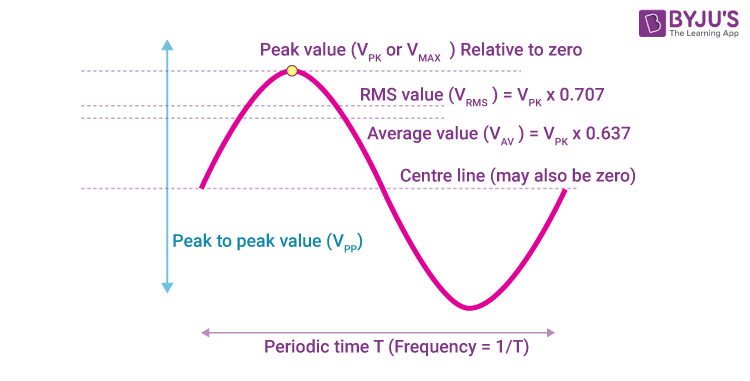# Peak and R.M.S Value of an Alternating Current / Voltage

The maximum value reached by an alternating quantity in one cycle is known as the peak value. This article let us know in detail about alternating current, the RMS value of alternating current, the RMS value of the AC formula, and the RMS value of alternating current derivation.

## What Is an Alternating Current?

Alternating current is defined as the current whose magnitude changes with time and also reverses its direction periodically.

The general equation is given by,

$$\begin{array}{l}I = I_o ~sin~ ωt\end{array}$$
$$\begin{array}{l}I = I_o ~cos~ ωt\end{array}$$

Where, Io is termed as peak value of an alternating current.Considering the above equation the current changes at any instantaneous time, if the current is passed through the electric circuit and it can be assumed to remain constant for any small time dt. As current is passed for a short time a small amount of charge is flown through the circuit in time dt and it is represented as:

If the current I is indicated as a sine function then,

$$\begin{array}{l}I =I_o ~sin~ ωt\end{array}$$
$$\begin{array}{l}dq=I ~dt\end{array}$$
$$\begin{array}{l}dq=I_o~ sin~ ωt~dt\end{array}$$

At half the period of an alternating current, the amount of charge passed through the circuit at time

T/2 is given by:

$$\begin{array}{l}q =\int\limits_{0}^{\frac{T}{2}} I_0 sin~ωt~dt\end{array}$$
$$\begin{array}{l}q = I_0 \int\limits_{0}^{\frac{T}{2}} sin~ωt~dt\end{array}$$
$$\begin{array}{l}q= I_0 [\frac{-cos ~ωt}{ω}]_{0}^{\frac{T}{2}}\end{array}$$
$$\begin{array}{l}q =\frac{-I_0}{ω} [cos~ωt]_{0}^{\frac{T}{2}}\end{array}$$
$$\begin{array}{l}q = \frac{-I_0 ~T}{2\pi} [cos~\pi ~-~ cos~0]\end{array}$$
$$\begin{array}{l}q = \frac{-I_0~T}{2\pi}[-1 -1]\end{array}$$
$$\begin{array}{l}q = \frac{I_0~T}{\pi}……….equation (1)\end{array}$$

The mean value of the alternating current is given by,

$$\begin{array}{l}q = I_m. ~\frac{T}{2} ……..equation (2)\end{array}$$

On equating equation (1) and equation (2), we get:

$$\begin{array}{l}I_m.~\frac{T}{2} = \frac{I_0~T}{\pi}\end{array}$$
$$\begin{array}{l}I_m = \frac{2I_0}{\pi} = 0.636~ I_0\end{array}$$

#### Do you know?

What will happen to the mean square value of an alternating current when it completes its full cycle?

The mean value of alternating current for completing the full cycle will be zero.

## RMS Value of Alternating Current

RMS stands for Root-Mean-Square of instantaneous current values. The RMS value of alternating current is given by direct current which flows through a resistance. The RMS value of AC is greater than the average value. The RMS value of sine current wave can be determined by the area covered in half-cycle. This is applicable to all the waves which include sinusoidal, non-sinusoidal, symmetrical, and asymmetrical. It is denoted by Irms or Iv.

## RMS Value of AC Formula

Following is the formula of RMS value of AC:

 $$\begin{array}{l}I_{r.m.s} = \frac{I_0}{√2}= 0.707 ~{I_0}\end{array}$$

## RMS Value of AC Derivation

$$\begin{array}{l}I= I_o~ sin~ ωt\end{array}$$
$$\begin{array}{l}d = I^2~ R~dt\end{array}$$
$$\begin{array}{l}dH = (I_0~sin~ωt)^2~R~dt\end{array}$$
$$\begin{array}{l}dH = I_0^2~R~sin^2~ωt~dt\end{array}$$

The heat produced in a time T/2 is given by:

$$\begin{array}{l}H = \int\limits_{0}^{\frac{T}{2}}~{I_0}^2~R~sin^2~ωt~dt\end{array}$$
$$\begin{array}{l}H ={I_0}^2~R~\int\limits_{0}^{\frac{T}{2}} sin^2~ωt~dt\end{array}$$
$$\begin{array}{l}H = \frac{{I_0}^2~R}{2}[\frac{T}{2}~-~0] = \frac{{I_0}^2~R}{2}.\frac{T}{2}……equation (3)\end{array}$$

The rms value of AC is represented as:

$$\begin{array}{l}H ={I_{r.m.s}}^2~R.~\frac{T}{2}………equation (4)\end{array}$$

By equating equation (3) and equation (4), we get:

$$\begin{array}{l}{I_{r.m.s}^2}~R.~\frac{T}{2}= \frac{{I_0}^2 ~R}{2}. \frac{T}{2}\end{array}$$
$$\begin{array}{l}{I_{r.m.s}^2}= \frac{{I_0}^2}{2}\end{array}$$
$$\begin{array}{l}I_{r.m.s} = \frac{I_0}{√2} = 0.707 ~{I_0}\end{array}$$

These values are measured by an ammeter and voltmeter that are used in the circuit.

Read More: Difference between Ammeter and Voltmeter

## Frequently Asked Questions – FAQs

Q1

### What is peak value?

Peak value is defined as the maximum value reached by an alternating quantity in one cycle is known as Peak value.

Q2

### What is meant by avarage value?

The average of all the instantaneous values of an alternating voltage and currents over one complete cycle gives the average Value.

Q3

### What is RMS value?

The square root of means of squares of instantaneous values gives the rms value.

Q4

### Which methods helps to determine the RMS values?

Methods used to determine rms value are:
• Graphical method
• Analytical method
Q5

### Energy transfer capability of an AC source can be calculated through which parameter?

Root Mean Square value (RMS value).

Register to “BYJU’S – The Learning App” for loads of interactive, engaging information and fall in love with learning.

Test your knowledge on Rms Value Alternating Current